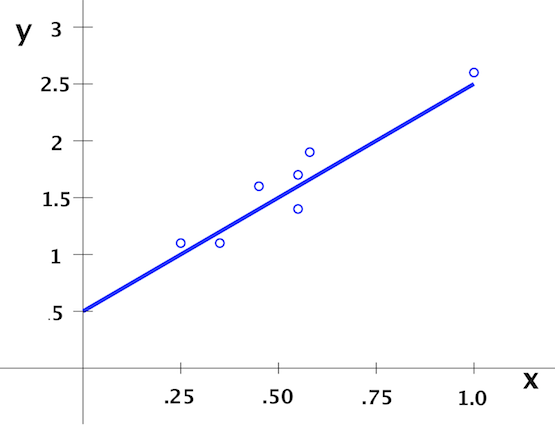# Regression & Correlation Flashcards

Regression & Correlation Flashcards
1/21 (missed) 0 0
Create Your Account To Continue Studying

As a member, you'll also get unlimited access to over 79,000 lessons in math, English, science, history, and more. Plus, get practice tests, quizzes, and personalized coaching to help you succeed.

Try it risk-free for 30 days. Cancel anytime
Negative Correlation

The regression line has a negative slope: when x values increase, the corresponding y values decrease and r, the correlation coefficient, is negative.

Got it
Positive Correlation

The slope of the regression line is positive: as the x values increase, the y values also increase and the correlation coefficient, r, is positive.

Got it
Causation

One event is the cause of another event.

Got it
Correlation

This is when a relationship exists between two events, but one event does not cause the other.

Got it
Normality

This assumes that the residuals follow a normal distribution around the line of best fit.

Got it
Homoscedasticity

This assumes that around the regression line, the variance in the values of the independent variable is the same.

Got it
Linearity

This assumes that there is a linear relationship between the independent and dependent variables.

Got it
Statistical Independence

In this assumption, the residuals vary randomly and do not follow a pattern.

Got it
Regression Analysis

A process for studying how variables are related in a statistical sense.

Got it
Residual

What you get when you find the difference between predicted and observed values.

Got it

or choose a specific lesson: See all lessons in this chapter
21 cards in set

## Flashcard Content Overview

This lesson covers the statistical concepts of correlation and causality as encountered when performing a regression analysis on a set of data. Skills covered are how to determine:

• the slope of the regression line
• the intercept of the regression line
• the correlation coefficient

Key terms include residual, statistical independence, linearity, homoscedasticity, negative correlation, positive correlation, variance and normality.

Front
Back
Residual

What you get when you find the difference between predicted and observed values.

Regression Analysis

A process for studying how variables are related in a statistical sense.

Statistical Independence

In this assumption, the residuals vary randomly and do not follow a pattern.

Linearity

This assumes that there is a linear relationship between the independent and dependent variables.

Homoscedasticity

This assumes that around the regression line, the variance in the values of the independent variable is the same.

Normality

This assumes that the residuals follow a normal distribution around the line of best fit.

Correlation

This is when a relationship exists between two events, but one event does not cause the other.

Causation

One event is the cause of another event.

Positive Correlation

The slope of the regression line is positive: as the x values increase, the y values also increase and the correlation coefficient, r, is positive.

Negative Correlation

The regression line has a negative slope: when x values increase, the corresponding y values decrease and r, the correlation coefficient, is negative.

r = 0

The variables are not correlated.

A small absolute value of r

The variables are weakly correlated.

Absolute value of r is close to 1

The variables are strongly correlated.

A battery's voltage increases by 0.1 volt for each hour of charging. x is the charging time in hours, and y is the voltage. When x is 0, y = 8. Write the linear equation for y in terms of x.

y = 0.1x + 8

where x is the charging time in hours and y is the voltage

A battery is charged at a rate of 0.5 volt per hour. Use x = hours and y = volts. At x = 0, y = 8. This battery's voltage should not exceed 12 volts. When should the charger be turned off?

y = 0.5x + 8, where x is the time in hours and y is the voltage. At x = 8 hours, the charger should be turned off because y will be equal to 12.

The cost to hire a consultant is \$100 plus \$200 for each hour of time on the job. Let the hours of time on the job be x and let y be the cost in dollars. Write a linear equation relating x and y.

y = 200x + 100

x = hours building a model, y = model cost. For x = 1, 2 and 3, y = 2, 4 and 8. The data is: (1, 2), (2, 4) and (3, 8). Use the formula for slope, a, and intercept, b, to find the linear model.

y = 3x - 4/3

First make your chart with columns for x, y, xy, and x^2 and find the sum of each column. Use the formulas for a and b.

a = [3(34) - 6(14)] / [3(14) - 6^2] = 18/6 = 3

b = 1/3[14 - 3(6)] = -4/3

The waiting time in days, y, for delivery of a gift is related to the amount, x, paid for postage. This data is observed: (10, 15), (20, 10) and (30, 5). Calculate the correlation coefficient, r.

r = -1

Scatter Plot

A plot of x-y ordered pairs showing how the data in x and y are related.

x is rainfall; y is growth. Intercept is?The intercept is 0.5. With no rainfall, the growth is 0.5 inches.

x = discount rate; y = sales. What is slope?Slope = 2. For each 0.25 change in discount rate, there will be a 0.5 increase in the number of sales.

To unlock this flashcard set you must be a Study.com Member.